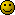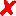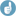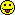Nav Coelho
●

2007-07-20 18:14
(5917 d 22:28 ago)

Posting: # 916
Views: 24,913

## Sample size estimation for parallel design [Power / Sample Size]

Dear all,

I have data from a parallel pilot study and am using that to estimate the sample size for a pivotal study. The formula that I am using requires an estimate of the inter subject variability which I don't have. I have the total variability (sqrt(MSE)). Is there a way to estimate intersubject variability from a parallel design or would it be correct to use the total variability instead?

Would it be correct to estimate inter-subject var as sd/mean for test and reference separately and then take the average?

And one last question, how correct is it to take 60% of inter subject (calculated as sd/mean) to be the intrasubject variability?

Nav Coelho
Helmut
★★★Vienna, Austria,
2007-07-20 18:29
(5917 d 22:13 ago)

@ Nav Coelho
Posting: # 917
Views: 22,210

## Parallel designs (total variance)

Dear Nav!

❝ I have data from a parallel pilot study and am using that to estimate the sample size for a pivotal study. The formula that I am using requires an estimate of the inter subject variability which I don't have. I have the total variability (sqrt(MSE)). Is there a way to estimate intersubject variability from a parallel design or would it be correct to use the total variability instead?

Hhm, yes you have the total variability (inter- + intra-subjects); the same will be true for your pivotal study. I don’t get your point of trying to estimate inter-subject variability from total. Which formula are you referring to?

❝ Would it be correct to estimate inter-subject var as sd/mean for test and reference separately and then take the average?

❝ And one last question, how correct is it to take 60% of inter subject (calculated as sd/mean) to be the intrasubject variability?

I don’t think so (both questions).

For an example please have a look at
Chow SC, Wang H. On Sample Size Calculation in Bioequivalence Trials. J Pharmacokin Pharmacodyn. 2001;28(2):155-69.
and Errata given at
J Pharmacokin Pharmacodyn. 2002;29(1):101-2.

You will also need two letters to the editor:
Hauschke D. A Note on Sample Size Calculation in Bioequivalence Trials. J Pharmacokin Pharmacodyn. 2002;29(1):89-94.
Blood P. Sample Size Calculation in Bioeqivalence Trials. J Pharmacokin Pharmacodyn. 2002;29(1):95-7.
H Wang H, SC Chow SC. Authors’ Response. J Pharmacokin Pharmacodyn. 2002;29(1):99.

Dif-tor heh smusma 🖖🏼 Довге життя Україна!Helmut SchützThe quality of responses received is directly proportional to the quality of the question asked. 🚮
Science Quotes
Nav Coelho
●

2007-07-20 20:38
(5917 d 20:05 ago)

(edited by Jaime_R on 2007-07-20 22:51)
@ Helmut
Posting: # 918
Views: 22,181

## Parallel designs (total variance)

Well, I am not sure how to estimate the sample size for a parallel design? The formula that I am using uses inter-subject variability and the reference is attached (Tutorial in Biostatisitcs: Sample sizes for clinical trials with Normal data by Steven A. Julious). Formula is on page 1970 #69. But how would you get the inter-subject variability from the ANOVA for parallel design in order to calculate the sample size. Thanks for the reference, I'll take a look.

Re: 60% of inter to estimate the intra: I am a statistician and I routinely see the PK individuals taking 60% of inter to be the intra for estimating sample size for a crossover design and always wondered about the validity of the approach.

Nav

--
Edit: Full quote removed. Please see this post! [Jaime]
Helmut
★★★Vienna, Austria,
2007-07-22 03:17
(5916 d 13:25 ago)

@ Nav Coelho
Posting: # 921
Views: 22,513

## CV-intra # 60% CV-inter

Dear Nav,

I’m not in my office, so be a little patient to get a thorough response – first I have to read your 66 pages of statistics.Just for completeness (because you sent me the paper by private mail):

Julious SA. TUTORIAL IN BIOSTATISTICS. Sample sizes for clinical trials with Normal data.
Stat Med. 2004;23(12):1921–86. doi:10.1002/sim.1783.

❝ Well, I am not sure how to estimate the sample size for a parallel design?

We’ll handle that…❝ Re: 60% of inter to estimate the intra: I am a statistician and I routinely see the PK individuals taking 60% of inter to be the intra for estimating sample size for a crossover design and always wondered about the validity of the approach.

Hey, that’s black magick!
Examples
1. Imagine two studies; one with very tight inclusion criteria with respect to anthrompometric covariates (sex, BMI, age, …), the other one very ‘liberal’. CVinter (but not CVintra!) will be higher in the first one; just my 2 ¢.
2. If I remember it correctly, diltiazem is a compound with low to moderate intra-subject CV (about 15%), but much higher CVinter.
3. Extreme examples are all studies employing different phenotypes. Whereas the phenotype is time-invariant – we don’t change our genotype in the washout – the intra-subject variability may be horrible (20fold differences in Cmax are not uncommon).
Lessons from the examples:
1. Although you may have established some kind of intra-/inter-CV ratio, this most likely is not – only – a property of the compound / formulation(s), but also of the study’s design/performance.
2. No, not 60%, rather 25%…
3. Again from my aging memory: buspirone CVintra ~15%, CVinter >80%?
Therefore I would consider

❝ 60% of inter to estimate the intra

again as black magick.Dif-tor heh smusma 🖖🏼 Довге життя Україна!Helmut SchützThe quality of responses received is directly proportional to the quality of the question asked. 🚮
Science Quotes
Helmut
★★★Vienna, Austria,
2007-07-23 19:44
(5914 d 20:59 ago)

@ Nav Coelho
Posting: # 925
Views: 25,557

## total (pooled) variance: examples

Dear Nav!

❝ […] But how would you get the inter-subject variability from the ANOVA for parallel design in order to calculate the sample size.

Some more references and explanations.
In a parallel design we have no direct access to inter-subject variability, what we see is the total (sometimes called pooled) variability.

From a 2×2×2 crossover design we may estimate
• intra-subject variability as
$$CV_e(\%)=100\sqrt{e^{MS_e}-1}$$

• inter-subject variability as
$$CV_s(\%)=100\sqrt{e^{(MS_s{\color{Red} -}ME_e)/2}-1}$$

• total (pooled) variability as
$$CV_p(\%)=100\sqrt{e^{(MS_s{\color{Red} +}ME_e)/2}-1}$$
For details see
1. Midha KK, Ormsby ED, Hubbard JW, McKay G, Hawes EM, Gavalas L, McGilveray IJ. Logarithmic Transformation in Bioequivalence: Application with Two Formulations of Perphenazine. J Pharm Sci. 1993;82(2):138–44.
2. Hauschke D, Steinijans VW, Diletti E. Presentation of the intrasubject coefficient of variation for sample size planning in bioequivalence studies. Int J Clin Pharmacol Ther. 1994;32(7):376–8.
So if you only have data from a parallel study, your variability is the total (pooled) one, not the inter-subject variability. People dealing with parallel studies sloppily talk about inter-subject variability instead of total.1 So I would guess you may simply use formulas and software intended for inter-subject CV (although you have the total CV). At least it’s a conservative approach (in other words, you will only loose some money because your study will be slightly overpowered)

❝ Re: 60% of inter to estimate the intra: I am a statistician and I routinely see the PK individuals taking 60% of inter to be the intra for estimating sample size for a crossover design and always wondered about the validity of the approach.

I give you two examples from my 2×2 cross-over studies (for low/medium/high intra-subject CV) to get an impression about the lacking relationship of CV-intra/-inter/-total:2

Methyphenidate 20 mg MR single dose sprinkled, AUCt, n=12
CV-intra  7.00% [=36% of inter, 34% of total]CV-inter 19.1%
CV-total 20.4%

Paroxetine 30 mg IR steady state, AUCtau, n=32
CV-intra 25.2% [=46% of inter, 41% of total]CV-inter 55.1%
CV-total 62.1%

Lansoprazole 30 mg single dose fasting, Cmax, n=47
CV-intra 47.0% [=187% (!) of inter, 86% of total]CV-inter 25.1%
CV-total 54.6%

I can’t see this rule of thumb of ‘CV-intra = 60% CV-inter’ to be applicable …

1. Intra-subject variability does not ‘disappear’ just because you made only one observation.

Dif-tor heh smusma 🖖🏼 Довге життя Україна!Helmut SchützThe quality of responses received is directly proportional to the quality of the question asked. 🚮
Science Quotes
Nav Coelho
●

2007-07-23 21:58
(5914 d 18:44 ago)

@ Helmut
Posting: # 927
Views: 22,027

## total (pooled) variance: examples

Dear Helmut,

I really appreciate you taking the time to answer my questions in such detail. In regards to the sample size calculation for a parallel design, I agree that the worst case scenario for taking total CV as the estimate for inter CV would only result in slight overestimation in power. Thanks for the confirmation!!

As for the 60% rule, you are absolutely right. It's not a one size fits all.

Nav

Edit: Full quote removed. Please see this post! [Helmut]
BEQool
☆

Slovenia,
2023-04-18 11:59
(167 d 04:43 ago)

@ Helmut
Posting: # 23525
Views: 1,471

## total (pooled) variance: examples

Hello!

It has been a long time since this was posted but I have been looking for ways to calculate inter-subject CV and I saw these following equations:

❝ […] ❝ From a 2×2×2 crossover design we may estimate

• intra-subject variability as

❝ $$CV_e(\%)=100\sqrt{e^{MS_e}-1}$$

• inter-subject variability as

❝ $$CV_s(\%)=100\sqrt{e^{(MS_s{\color{Red} -}ME_e)/2}-1}$$

• total (pooled) variability as

❝ $$CV_p(\%)=100\sqrt{e^{(MS_s{\color{Red} +}ME_e)/2}-1}$$

And since it was mentioned that we may estimate CVs with these equations from a 2x2x2 crossover study I am curious if this equations for CVs also apply for other higher order designs?

Best regards
BEQool
Helmut
★★★Vienna, Austria,
2023-04-20 12:19
(165 d 04:23 ago)

@ BEQool
Posting: # 23530
Views: 1,396

## Higher-Order Crossovers

Hi BEQool,

❝ ❝ ❝ From a 2×2×2 crossover design we may estimate❝ And since it was mentioned that we may estimate CVs with these equations from a 2x2x2 crossover study I am curious if this equations for CVs also apply for other higher order designs?

Yes. I strongly suggest to follow the ‘Two at a Time’-approach instead of an ANOVA of pooled data (see there).

Dif-tor heh smusma 🖖🏼 Довге життя Україна!Helmut SchützThe quality of responses received is directly proportional to the quality of the question asked. 🚮
Science Quotes
BEQool
☆

Slovenia,
2023-04-21 09:53
(164 d 06:49 ago)

@ Helmut
Posting: # 23532
Views: 1,370

## Higher-Order Crossovers

Great, thank you!
zizou
★

Plzeň, Czech Republic,
2023-04-21 23:23
(163 d 17:20 ago)

(edited by on 2023-04-22 00:24)
@ BEQool
Posting: # 23536
Views: 1,277

## total (pooled) variance: examples

Dear BEQool,
yes for intra-subject CV and
no for inter/total-subject CVs in general.

The equations stated above are valid for 2-treatment crossover designs.

However e.g. for a 3×6×3 crossover design, i.e. with 3 treatments (R1R2T or pilot T1T2R), we may estimate
• intra-subject variability as
$$CV_e(\%)=100\sqrt{e^{MS_e}-1}$$
• inter-subject variability as
$$CV_s(\%)=100\sqrt{e^{(MS_s{-}MS_e)/\color{Blue} 3}-1}$$
• total (pooled) variability as
$$CV_p(\%)=100\sqrt{e^{(MS_s{+}MS_e)/\color{Blue} 3}-1}$$
Best regards,
zizou
BEQool
☆

Slovenia,
2023-04-22 20:20
(162 d 20:23 ago)

@ zizou
Posting: # 23537
Views: 1,266

## total (pooled) variance: examples

Thank you zizou for the explanation.

So if we have e.g. 3x6x3 Williams crossover design (with 3 treatments), the number in the denominator depends on the approach we use?
If we use "Two at a Time" approach with incomplete block designs, we are only evaluating 2 treatments and therefore we should use number 2 in the denominator; but if we use "All at Once" approach, we are evaluating all 3 treatments and we have to use number 3 in the denominator?

Additional question (just to be sure): we also use number 2 in the denominator (to get inter-subject variance) when we have replicate designs with 2 treatments (regradless of the partial- or full-replicate design)?
zizou
★

Plzeň, Czech Republic,
2023-04-22 23:06
(162 d 17:36 ago)

(edited by on 2023-04-22 23:40)
@ BEQool
Posting: # 23538
Views: 1,238

## total (pooled) variance: examples

Dear BEQool.

❝ So if we have e.g. 3x6x3 Williams crossover design (with 3 treatments), the number in the denominator depends on the approach we use?

No, for both approaches the denominator is 3. It's not affected by whether the model is complete or incomplete.
Nevertheless someone else could check it. I read about it many years ago (mentioned here and statistical background somewhere else, I don't remember where). And "a long time ago in a galaxy far, far away" I also recalculated the estimate (obtained from ANOVA - GLM and equation with 3 as denominator) using the mixed model where the inter-subject variability is obtained more directly.

❝ If we use "Two at a Time" approach with incomplete block designs, we are only evaluating 2 treatments and therefore we should use number 2 in the denominator; but if we use "All at Once" approach, we are evaluating all 3 treatments and we have to use number 3 in the denominator?

As Helmut mentioned, the "Two at a Time" is preffered approach. In the "All at Once" analysis, 90% confidence intervals in comparison of T and R1 are (slightly) affected by values of R2 and vice versa. It is not desirable in any of comparisons (e.g. when R1 is from EU and R2 from USA).
So: "..., the analysis for each comparison should be conducted excluding the data from the treatments that are not relevant for the comparison in question." (EMA Guideline 1401)

❝ Additional question (just to be sure): we also use number 2 in the denominator (to get inter-subject variance) when we have replicate designs with 2 treatments (regradless of the partial- or full-replicate design)?

Yes. At least I hope so, I have experience only with full replicate designs so far.
BEQool
☆

Slovenia,
2023-04-25 12:57
(160 d 03:45 ago)

@ zizou
Posting: # 23539
Views: 1,134

## total (pooled) variance: examples

Dear zizou,

❝ No, for both approaches the denominator is 3. It's not affected by whether the model is complete or incomplete. Nevertheless someone else could check it. I read about it many years ago (mentioned here and statistical background somewhere else, I don't remember where). And "a long time ago in a galaxy far, far away" I also recalculated the estimate (obtained from ANOVA - GLM and equation with 3 as denominator) using the mixed model where the inter-subject variability is obtained more directly.

I have checked it nowI made up (Cmax) concentrations for 3x6x3 Williams design (with treatments A,B,C) with 18 subjects (complete dataset, all subjects completed all periods) and run it with Phoenix WNL.
Here are the results:

a) All at once approach

Inter-subject CV given directly with mixed model approach = 13.4 %
Inter-subject CV calculated from ANOVA table with denominator 3 = 13.4 % (right)
Inter-subject CV calculated from ANOVA table with denominator 2 = 16.5 % (wrong)

So here when using (wrong) All at once approach, the number in the denominator should be the same as the total number of treatments (the number of all treatments we are evaluating)

b) Two at a Time approach (incomplete block design); B vs. C

Inter-subject CV given directly with mixed model approach = 6.6 %
Inter-subject CV calculated from ANOVA table with denominator 3 = 5.4 % (wrong)
Inter-subject CV calculated from ANOVA table with denominator 2 = 6.6 % (right)

But here when using Two at a Time approach (where we are evaluating just 2 treatments), it seems that we have to use number 2 in the denominator. So it seems that the denominator is affected by whether the model is complete or incomplete.

c) Two at a Time approach (incomplete block design); A vs. C

Here we should of course as above also probably use number 2 in the denominator but here interestingly something else happens:
Inter-subject CV given directly with mixed model approach = ERROR
Inter-subject CV calculated from ANOVA table with denominator 3 = ERROR
Inter-subject CV calculated from ANOVA table with denominator 2 = ERROR

Even when using Subject as fixed effect (which is the solution for such cases according to Certara) MSE (0.22973) is larger than MSS (0.20599), therefore inter-subject variance is negative (-0.0119; the same number is given directly with mixed model). Therefore the calculation of inter-subject CV is impossible (error).

Why is this so and what should be done in this case? This probably happens because intra-subject CV is so much higher than inter-subject CV? I know these data that I used is made up but nevertheless it could happen with real-world data as well?
zizou
★

Plzeň, Czech Republic,
2023-04-26 00:43
(159 d 15:59 ago)

(edited by on 2023-04-26 19:05)
@ BEQool
Posting: # 23540
Views: 1,104

## total (pooled) variance: examples

Dear BEQool, well done!❝ So it seems that the denominator is affected by whether the model is complete or incomplete.

Thank you for correcting me. I didn’t remember it correctly. I was sure about denominator equal to 3 in “All at Once” approach (i.e. one ANOVA). The another (better) approach I didn’t use for past several years and I forgot the details like the denominator used. Sorry for the confusion. So it seems the denominator is equal to the number of treatments in evaluation.Btw. total (pooled) CV in 2x2x2 can also be obtained by fitting the model with factors Formulations, Periods, Sequences (i.e. without Subjects(Sequences)). Then use MSE in equation:
CV=sqrt(e^(MSE)-1) (multiplicative model with residual variance sigma^2 which is estimated by MSE from ANOVA)
For parallel design, it's trivial and only the total (pooled) CV can be obtained.
For higher-order designs with more than two formulations I never tried it but it could be another option for recalculation.

❝ ... it could happen with real-world data as well?

Sometimes (not often) it happens. It was discussed e.g. in this thread.
BEQool
☆

Slovenia,
2023-05-04 10:17
(151 d 06:26 ago)

@ zizou
Posting: # 23542
Views: 795

## total (pooled) variance: examples

❝ Btw. total (pooled) CV in 2x2x2 can also be obtained by fitting the model with factors Formulations, Periods, Sequences (i.e. without Subjects(Sequences)). Then use MSE in equation:

CV=sqrt(e^(MSE)-1) (multiplicative model with residual variance sigma^2 which is estimated by MSE from ANOVA)

❝ For parallel design, it's trivial and only the total (pooled) CV can be obtained.

Interesting, thanks!

❝ For higher-order designs with more than two formulations I never tried it but it could be another option for recalculation.

Just for curiosity, with above mentioned data I tried some calculations for 3x6x3 design with and without factor Subject(Sequence) for both approaches (All at once and Two at a time) but I couldnt get matched CVtotal numbers so like you said there is probably another option for recalculation.

❝ ❝ ... it could happen with real-world data as well?

❝ Sometimes (not often) it happens. It was discussed e.g. in this thread.

Thanks!
Dipesh Jayswal
●

2007-07-24 10:52
(5914 d 05:50 ago)

@ Nav Coelho
Posting: # 928
Views: 21,955

## Sample size estimation for parallel design

Dear Nav Coelho,

The following article may help u out for determination of sample size assuming different variances of test and reference formulation which is recommanded by FDA.

"Hansheng Wang and Shein-Chung Chow, A practical approach for comparing means of two groups without equal variance assumption, Statistics in Medicine 2002; 21:3137–3151"Dipesh Jayswal

Edit: Online resource. [Helmut]Ing. Helmut Schütz